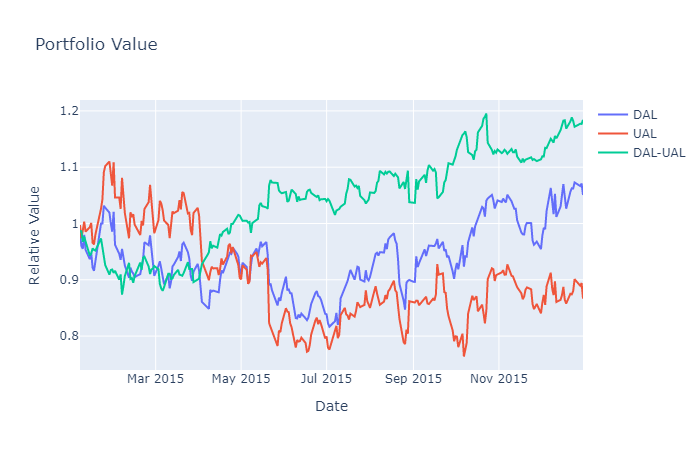# Datasets

## Equity Fundamental Data

### Introduction

This page explains how to request, manipulate, and visualize historical Equity Fundamental data.

### Prerequisites

Working knowledge of C#.

Working knowedge of Python and pandas. If you are not familiar with pandas, see the pandas documentation.

### Get Historical Data

Create subscriptions to all of the Equities for which you want fundamental historical data and then call the GetFundamental method with a list of Symbols, a required fundamental data field name string, start DateTimedatetime, and end DateTimedatetime to request fundamental historical data for those specific securities. To view the possible fundamental data field names, see the FineFundamental attributes in Data Point Attributes. For example, to get data for air companies over 2014, run:

var symbols = new []
{
"AAL",  // American Airlines Group, Inc.
"ALGT", // Allegiant Travel Company
"ALK",  // Alaska Air Group, Inc.
"DAL",  // Delta Air Lines, Inc.
"LUV",  // Southwest Airlines Company
"SKYW"  // SkyWest, Inc.
"UAL",  // United Air Lines
}

var startTime = new DateTime(2014, 1, 1);
var endTime = new DateTime(2015, 1, 1);
var peRatios  = qb.GetFundamental(symbols, "ValuationRatios.PERatio",
startTime, endTime);

symbols = [qb.AddEquity(ticker).Symbol
for ticker in [
"AAL",   # American Airlines Group, Inc.
"ALGT",  # Allegiant Travel Company
"ALK",   # Alaska Air Group, Inc.
"DAL",   # Delta Air Lines, Inc.
"LUV",   # Southwest Airlines Company
"SKYW"   # SkyWest, Inc.
"UAL",   # United Air Lines
]]

start_time = datetime(2014, 1, 1)
end_time = datetime(2015, 1, 1)
pe_ratios = qb.GetFundamental(symbols, "ValuationRatios.PERatio",
start_time, end_time)

The call returns the fundamental data field that are timestamped within the defined period of time.

The GetFundamental method returns a pandas.DataFrame where the index is the time and the columns are the Equity Symbol.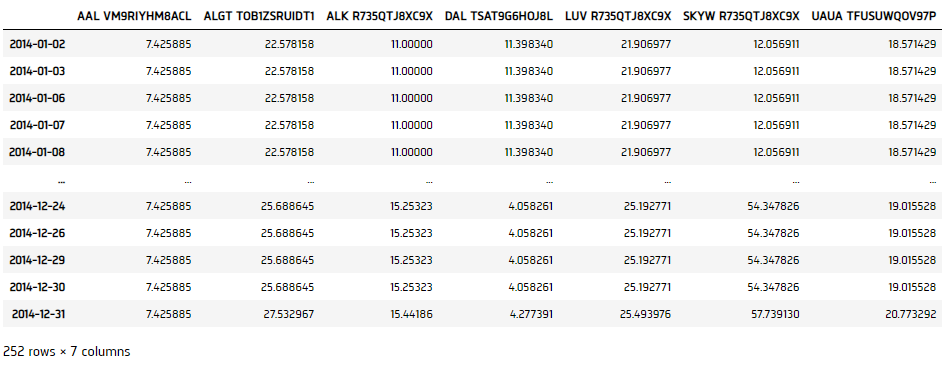The GetFundamental method returns an IEnumerable<DataDictionary> for multiple-security requests.

### Wrangle Data

You need a subscription before you can request historical data for a security. You can request an amount of historical data based on a defined period of time.

#### Select One Security

Index the DataFrame with a Symbol to select the historical data of the security.

// Take the first 10 slice as example.
foreach(var bar in ebitda.Select(data => (data[symbol], data.Time)).Take(10))
{
Console.WriteLine($"{bar}"); } # Reset the column names ebitda.columns = [col.split(" ") for col in ebitda.columns] # Select the column ebitda[symbol.Value]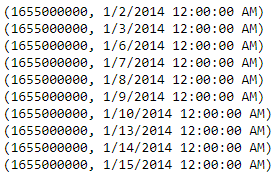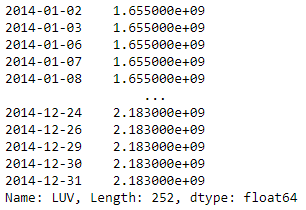#### Select By Mean We'd like to select the Symbols with the highest and lowest average PE Ratio. 1. Initialize a Dictionary to hold the data. 2. var meanPE = new Dictionary<Symbol, decimal>(); 3. Iterate each Symbol and calculate their mean, then add to the dictionary. 4. foreach(var sym in symbols){ var avg = peRatios.Select(data => (decimal) data[sym]).Average(); meanPE.Add(sym, avg); Console.WriteLine($"The Average PE Ratio of {sym}: {avg}");
}
5. Select the Symbol with highest and lowest mean.
6. var symbolWithMaxPE = meanPE.FirstOrDefault(x => x.Value == meanPE.Values.Max()).Key;
var symbolWithMinPE = meanPE.FirstOrDefault(x => x.Value == meanPE.Values.Min()).Key;
Console.WriteLine($"The Highest Average PE Ratio: {symbolWithMaxPE}: {meanPE[symbolWithMaxPE]}"); Console.WriteLine($"The Lowest Average PE Ratio: {symbolWithMinPE}: {meanPE[symbolWithMinPE]}");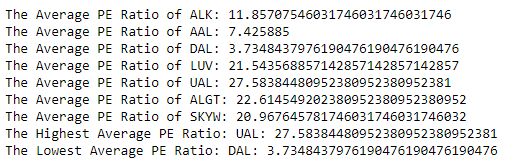1. Obtain the mean of each Symbol by calling mean method.
2. mean_pe = pe_ratios.mean()
3. Call sort_values to sort in ascending order.
4. sorted_by_mean_pe = pe_ratios.mean().sort_values()
5. Select the Symbol with highest and lowest mean.
6. highest_avg_pe = qb.Symbol(sorted_by_mean_pe.index[-1])
lowest_avg_pe = qb.Symbol(sorted_by_mean_pe.index)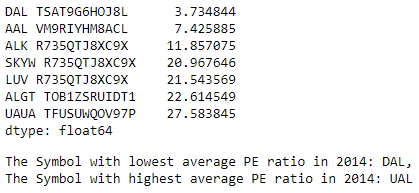#### Select By Percentage Change

We'd like to select the Symbols with the highest and lowest percentage change in the last PE Ratio.

1. Initialize a Dictionary to hold the data.
2. var peOnUpdate = new Dictionary<Symbol, List<decimal>>();
3. Iterate each Symbol and filter out the duplicates, then add to the dictionary.
4. foreach(var sym in symbols){
if (!peOnUpdate.ContainsKey(sym)){
peOnUpdate[sym] = new List<decimal>();
}
foreach(var bar in peRatios.Select(data => (decimal) data[sym])){
if (peOnUpdate[sym].Count() == 0 || bar != peOnUpdate[sym].Last()){
}
}
}
5. Calculate the last PE Ratio % change, save as a dictionary.
6. var lastPctChgPE = peOnUpdate.ToDictionary(kvp => kvp.Key,
kvp => kvp.Value.Count() > 2 ?
(kvp.Value.Last() - kvp.Value[kvp.Value.Count() - 2]) / kvp.Value[kvp.Value.Count() - 2] :
0m);
foreach(var kvp in lastPctChgPE){
Console.WriteLine($"The latest % change in PE Ratio of {kvp.Key}: {kvp.Value}"); } 7. Select the Symbols with highest and lowest % change. 8. var symbolWithMaxPEChg = lastPctChgPE.FirstOrDefault(x => x.Value == lastPctChgPE.Values.Max()).Key; var symbolWithMinPEChg = lastPctChgPE.FirstOrDefault(x => x.Value == lastPctChgPE.Values.Min()).Key; Console.WriteLine($"The Highest %Change PE Ratio: {symbolWithMaxPEChg}: {lastPctChgPE[symbolWithMaxPEChg]}");
Console.WriteLine(\$"The Lowest %Change PE Ratio: {symbolWithMinPEChg}: {lastPctChgPE[symbolWithMinPEChg]}");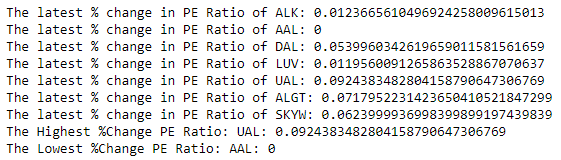1. Call drop_duplicates to retain the data points when the Fundamentals are updated.
2. pe_on_update = pe_ratios.drop_duplicates()
3. Call pct_change to get their percentage change in Fundamentals.
4. pe_pct_chg = pe_on_update.pct_change()
5. Get the latest data point by indexing -1.
6. latest_pe_pct_chg = pe_pct_chg.iloc[-1]
7. Call sort_values to sort in ascending order.
8. sorted_by_pe_pct_chg = latest_pe_pct_chg.sort_values()
9. Select the Symbols with highest and lowest % change.
10. highest_pe_pct_chg = qb.Symbol(sorted_by_pe_pct_chg.index[-1])
lowest_pe_pct_chg = qb.Symbol(sorted_by_pe_pct_chg.index)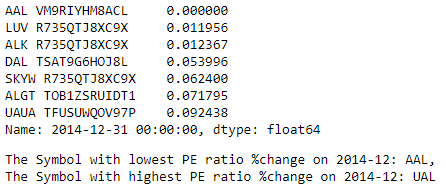### Plot Data

Jupyter Notebooks don't currently support libraries to plot historical data, but we are working on adding the functionality. Until we add the functionality, use Python to plot historical fundamental data.

You need a subscription before you can request historical data for a security. You can request an amount of historical data based on a defined period of time.

We'll use Plotly library for plotting.

# Import the plotly library.
import plotly.graph_objects as go

#### PE Ratio Over Time

We'd like to visualize how the PE Ratio evolve for airlines in 2014.

1. Call reset_index.
2. pe_cols = pe_ratios.columns
pe_ratios_ = pe_ratios.reset_index()
3. Plot the lines.
4. fig = go.Figure()
for i in range(len(pe_cols)):
y=pe_ratios_[pe_cols[i]],
name=pe_cols[i].split(" ")))
5. Set the title and axis labels.
6. fig.update_layout(title="PE Ratio Over Time",
xaxis_title="Date",
yaxis_title="PE Ratio")
7. Show the figure.
8. fig.show()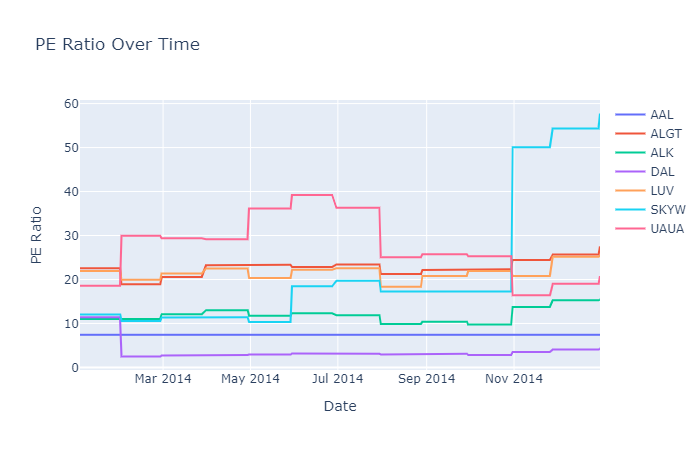We hypothesize that in the same sector, the relatively undervalued (lowest average PE Ratio) would out perform the relatively overvalued (higehest average PE Ratio) stock by market efficiency. We would visualize this for validation.

1. Get historical data for the forward year's (2015) price data for the highest and lowest average PE Ratio airlines.
2. history = qb.History([highest_avg_pe, lowest_avg_pe],
datetime(2015, 1, 1),
datetime(2015, 12, 31),
Resolution.Daily).close.unstack(level=0)
3. Calculate daily cumulative returns.
4. equity_value_over_time = (history.pct_change()[1:] + 1).cumprod()
5. Call reset_index.
6. equity_value_over_time = equity_value_over_time.reset_index()
7. Plot the lines.
8. fig = go.Figure()
y=equity_value_over_time[lowest_avg_pe],
name=str(lowest_avg_pe)))
y=equity_value_over_time[highest_avg_pe],
name=str(highest_avg_pe)))
name=f"{str(lowest_avg_pe)}-{str(highest_avg_pe)}"))
10. fig.update_layout(title="Portfolio Value",
yaxis_title="Relative Value")
12. fig.show()